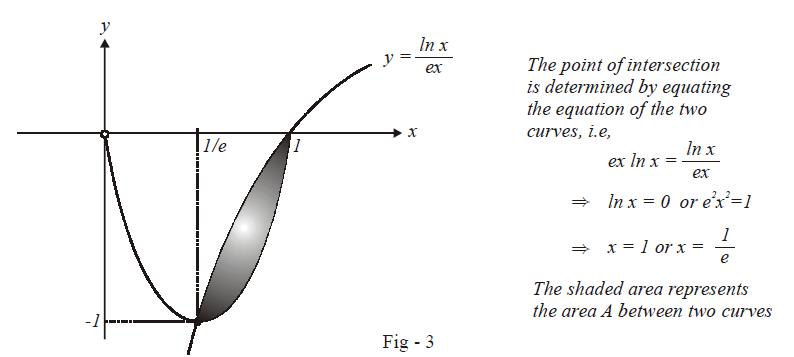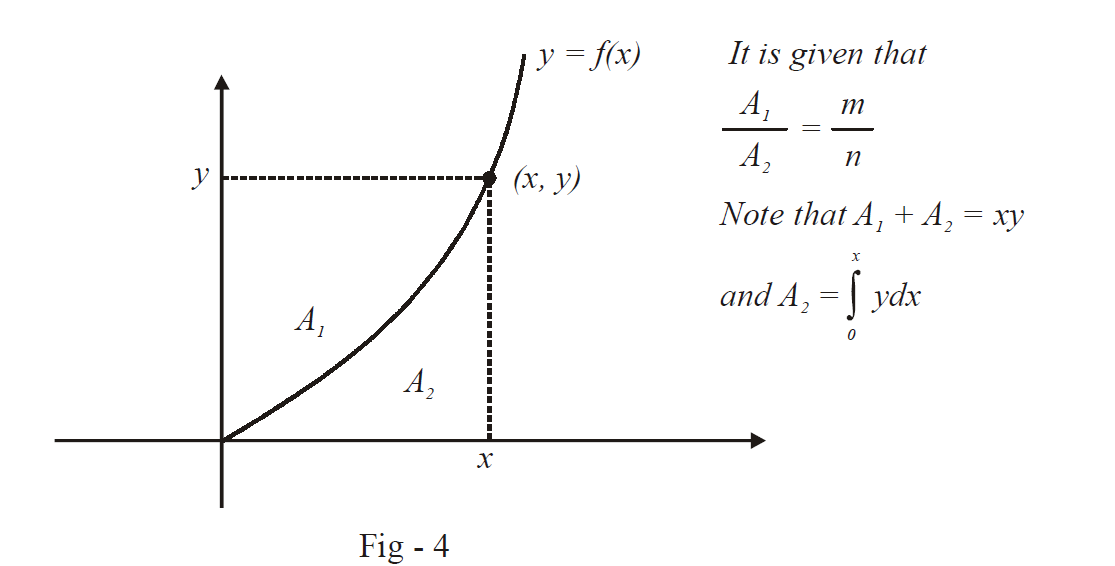# Problems On Areas Set-2

Go back to  'Areas and Volumes'

Example – 3

Compute the area of the region bounded between the curves $$y = ex\,\,\,\text{In}\,\,\,\,x\,\text{and}\,y =\frac{{Inx}}{{ex}}$$

Solution: If you’ve read the chapter on “Applicationsof Derivatives” properly, you should not have much of a problem plottingthe graph of the two functions:From the figure, it is clear that

\begin{align}A &= \int\limits_{1/e}^1 {\left( {\frac{{1n\,x}}{{ex}}- ex\,1n\,x} \right)} dx\\&= \,\int\limits_{1/e}^1{\frac{{1n\,x}}{{ex}}} dx - e\,\int\limits_{1/e}^1 {x\,1n\,\,\,xdx} \\&= \left\{ {\frac{1}{e}\int\limits_{ - 1}^0 {tdt} } \right\} - \left. {e\left\{{\frac{{{x^2}}}{2}1n\,x} \right.} \right|_{1/e}^1\left. { -\frac{1}{2}\int\limits_{1/e}^1 {xdx} } \right\}\\&= \frac{{ -1}}{{2e}} - e\left\{ {\frac{1}{{2{e^2}}} - \frac{1}{4}\left( {1 -\frac{1}{{{e^2}}}} \right)} \right\}\\&= - \frac{1}{{2e}} -\frac{1}{{2e}} + \frac{e}{4}\left( {\frac{{{e^2} - 1}}{{{e^2}}}} \right)\\&= - \frac{1}{e} + \frac{{{e^2} - 1}}{{4e}}\\&= \frac{{{e^2} -5}}{{4e}}\,\,sq.\,\rm{units}\end{align}

Example – 4

A curve $$y = f\left( x\right)$$ passes through the origin. Through any point $$\left( {x,{\rm{ }}y} \right)$$ on the curve,lines are drawn parallel to the co-ordinate axis. If the curve divides the areaformed by these lines and the co-ordinate axes in the ratio m:n, find theequation of the curve

Solution: The situation described in the question is graphically depicted below:From the given constraint, we have

\begin{align}&\,\,\,\,\,\,\,\frac{{xy -\int\limits_0^x {y\,dx} }}{{\int\limits_0^x {y\,dx} }} = \frac{m}{n}\\\\&\Rightarrow \int\limits_0^x {y\,dx = } \frac{n}{{m + n}}\left( {xy} \right)\end{align}

Differentiating both sides with respect to $$x,$$ we obtain

\begin{align}&\qquad \;\; y = \frac{n}{{m + n}}\left({\frac{{xdy}}{{dx}} + y} \right)\\\\&\Rightarrow \quad my = nx\frac{{dy}}{{dx}}\\\\&\Rightarrow \quad \frac{{dy}}{y} = \frac{m}{n}\frac{{dx}}{x}\\\\&\Rightarrow \quad 1n\,y =\,\frac{m}{n}1n\,x\, + c\\\\&\qquad \qquad = \frac{m}{n}1n\,x\, +1n\,k\,\,\,\,{\rm{where\;k\;is\;a\;constant}}\\\\& \Rightarrow \quad y =k{x^{m/n}}\,\,\,\,\,\,\,\,\,\,\,\,\,\,\,\,\,\,\,\,\,\,\,\,{\rm{(Note\;that\;this\;passes\;through\;the\;origin)}}\end{align}